#LOGARITHMS AND THEIR INVERSESIf the logarithmic function is one-to-one, its inverse exits. The inverse of a logarithmic function is an exponential function. When you graph both the logarithmic function and its inverse, and you also graph the line y = x, you will note that the graphs of the logarithmic function and the exponential function are mirror images of one another with respect to the line y = x. If you were to fold the graph along the line y = x and hold the paper up to a light, you would note that the two graphs are superimposed on one another. Another way of saying this is that a logarithmic function and its inverse are symmetrical with respect to the line y = x.

Work through the following examples.

Example 1: Find the inverse of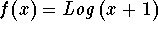.

Comments: Recall that the composition of a function with its inverse will take you back to where you started. For example, suppose the rule f(x) will take a 3 and link it to 10; then the rule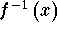will take the 10 and link it back to the 3. Another way of stating this is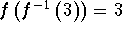. A general way to stating this is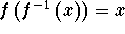for any x in the domain of f(x).

Solution: With respect to this problem,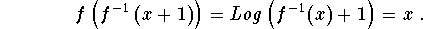The base is 10, the exponent is x, and the problem can be converted to the exponential function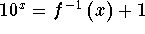which can be simplified to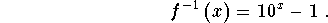Recall that the domain of f(x) is equal to the range of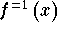, and the range of f(x) is equal to the domain of. The domain of f(x) is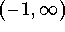and the range of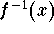is also. In terms of the graphs of these functions, this means that the entire graph of f(x) will be located to the right of the vertical line x = - 1, and the entire graph ofwill be located above the line y = - 1.

Let's check our answer by finding points on both graphs. In the original graph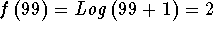. This means that the point (99, 2) is located on the graph of f(x). If we can show that the point (2, 99) is located on the inverse, we have shown that our answer is correct, at least for these two points.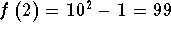indicates that the point (2, 99) is located on the graph of the inverse function. We have correctly calculated the inverse of the logarithmic function f(x). This is not the ``pure'' proof that you are correct; however, it works at an elementary level.

Example 2: Find the inverse of the function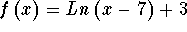.

Solution: Using the fact thatand the base is e, we can determine the inverse function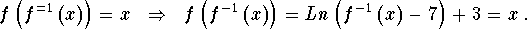Step 1:
We can convert this equation to an exponential equation of base e. First isolate the Ln term by subtracting 3 from each side.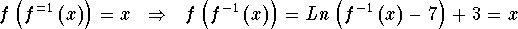can be written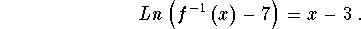Step 2:
Convert the above equation to an exponential equation with base e: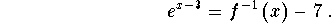Step 3:
When you add 7 to both sides we, theterm is isolated..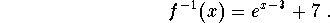Step 4:
Note that the domain of f(x) is the set of all real numbers greater than 7, and the range ofis also the set of all real numbers greater than 7.

Step 5:
You can check your answer by graphing both functions and determining whether they are symmetric to the line y = x. You can also calculate points. Suppose (8, 3) is a point on the graph of the original function, then if we can show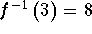; i.e. the point (3, 8) is located on the graph of the function, then odds are we have calculated the inverse correctly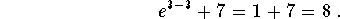Well Done.

If you would like to review another example, click on Example.

If you like to work a few problems and check the solutions, click on Answer below.

Problem 1: Find the inverse, if it exists, to the function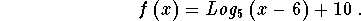If it does not exist, indicated the restricted domain where it will exist.

Problem 2: Find the inverse, if it exists, to the function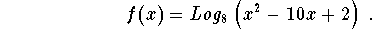If it does not exist, indicated the restricted domain where it will exist.

Problem 3: Find the inverse, if it exists, to the function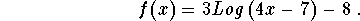If it does not exist, indicated the restricted domain where it will exist.[Back to Rules of Logarithms] [Back to Exponential Functions]

[Algebra] [Trigonometry] [Complex Variables]S.O.S MATHematics home page

Do you need more help? Please post your question on our S.O.S. Mathematics CyberBoard.Author: Nancy Marcus